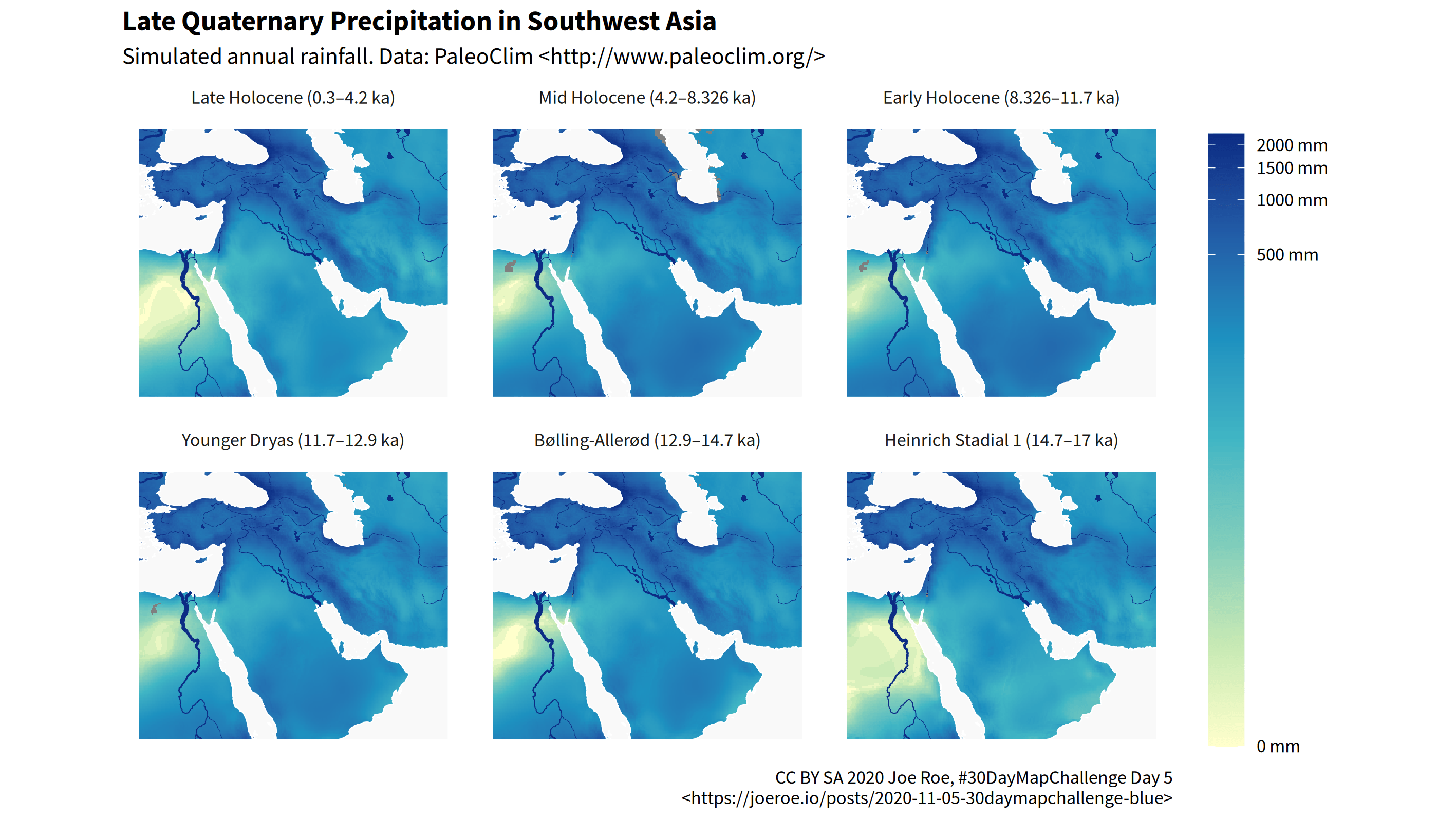[This article was first published on Joe Roe, and kindly contributed to R-bloggers]. (You can report issue about the content on this page here)
Want to share your content on R-bloggers? click here if you have a blog, or here if you don't.

The theme for day 5 of the #30DayMapChallenge is “blue”. My submission is a map of simulated rainfall in Southwest Asia during key climate periods in the Late Quaternary.The map was generated in R using the code below. View the RMarkdown source on GitHub.

```library("tidyverse")
library("sf")
library("raster")
library("stars")
library("glue")
library("rnaturalearth")
library("ragg")

# remotes::install_github("joeroe/rpaleoclim")
library("rpaleoclim")
```

## Data

I used reconstructed climate data from PaleoClim. My package rpaleoclim makes getting data from PaleoClim more straightforward.

```sw_asia <- extent(c(25, 65, 15, 45))

tribble(
~code, ~name,                ~start, ~end,
"lh",  "Late Holocene",      4.2,    0.3,
"mh",  "Mid Holocene",       8.326,  4.2,
"eh",  "Early Holocene",     11.7,   8.326,
"yds", "Younger Dryas",      12.9,   11.7,
"ba",  "Bølling-Allerød",    14.7,   12.9,
"hs1", "Heinrich Stadial 1", 17.0,   14.7
) %>%
mutate(paleoclim = map(code, paleoclim, region = sw_asia)) %>%
mutate(paleoclim = map(paleoclim, subset, subset = "bio_12")) ->
palclim
```

As always, Natural Earth provides the base layers.

```ocean <- ne_download(scale = 10, type = "ocean", category = "physical",
returnclass = "sf")
lakes <- ne_download(scale = 10, type = "lakes", category = "physical",
returnclass = "sf")
rivers <- ne_download(scale = 10, type = "rivers_lake_centerlines_scale_rank",
category = "physical", returnclass = "sf")

ocean <- st_make_valid(ocean)
ocean <- st_crop(ocean, sw_asia)
lakes <- st_crop(lakes, sw_asia)
rivers <- st_crop(rivers, sw_asia)
```

## Plot

I converted the palaeoclimate data from `Raster` to stars format, since the latter makes it easier to combine raster and `sf` vector layers.

```palclim\$paleoclim %>%
stack() %>%
st_as_stars() %>%
st_set_dimensions("band",
values = glue_data(palclim, "{name} ({end}–{start} ka)")) ->
palclim_cube

ggplot() +
geom_stars(data = palclim_cube) +
geom_sf(data = ocean, fill = "#f9f9f9", colour = "#ffffff", size = 0.25) +
geom_sf(data = rivers, mapping = aes(size = strokeweig / 2),
colour = "#0c2c84") +
geom_sf(data = lakes, fill = "#0c2c84", colour = NA) +
geom_sf(data = sw_asia %>% as("SpatialPolygons") %>% st_as_sf() %>% st_set_crs(4326),
fill = NA, colour = "#ffffff", size = 0.5) +
scale_fill_distiller(palette = "YlGnBu", direction = 1, trans = "pseudo_log",
labels = function(x) paste(x, "mm"),
guide = guide_colourbar(title = NULL,
frame.colour = "#ffffff",
ticks.colour = "#ffffff",
barheight = unit(0.75, "snpc"))) +
scale_size_identity() +
facet_wrap(~band) +
labs(
x = NULL, y = NULL,
title = "Late Quaternary Precipitation in Southwest Asia",
subtitle = "Simulated annual rainfall. Data: PaleoClim <http://www.paleoclim.org/>",
caption = paste("CC BY SA 2020 Joe Roe, #30DayMapChallenge Day 5",
"<https://joeroe.io/posts/2020-11-05-30daymapchallenge-blue>",
sep  = "\n")
) +
theme_minimal(
base_family = "Source Sans Pro",
) +
theme(
plot.title = element_text(face = "bold"),
axis.text = element_blank(),
panel.grid = element_blank(),
)
```